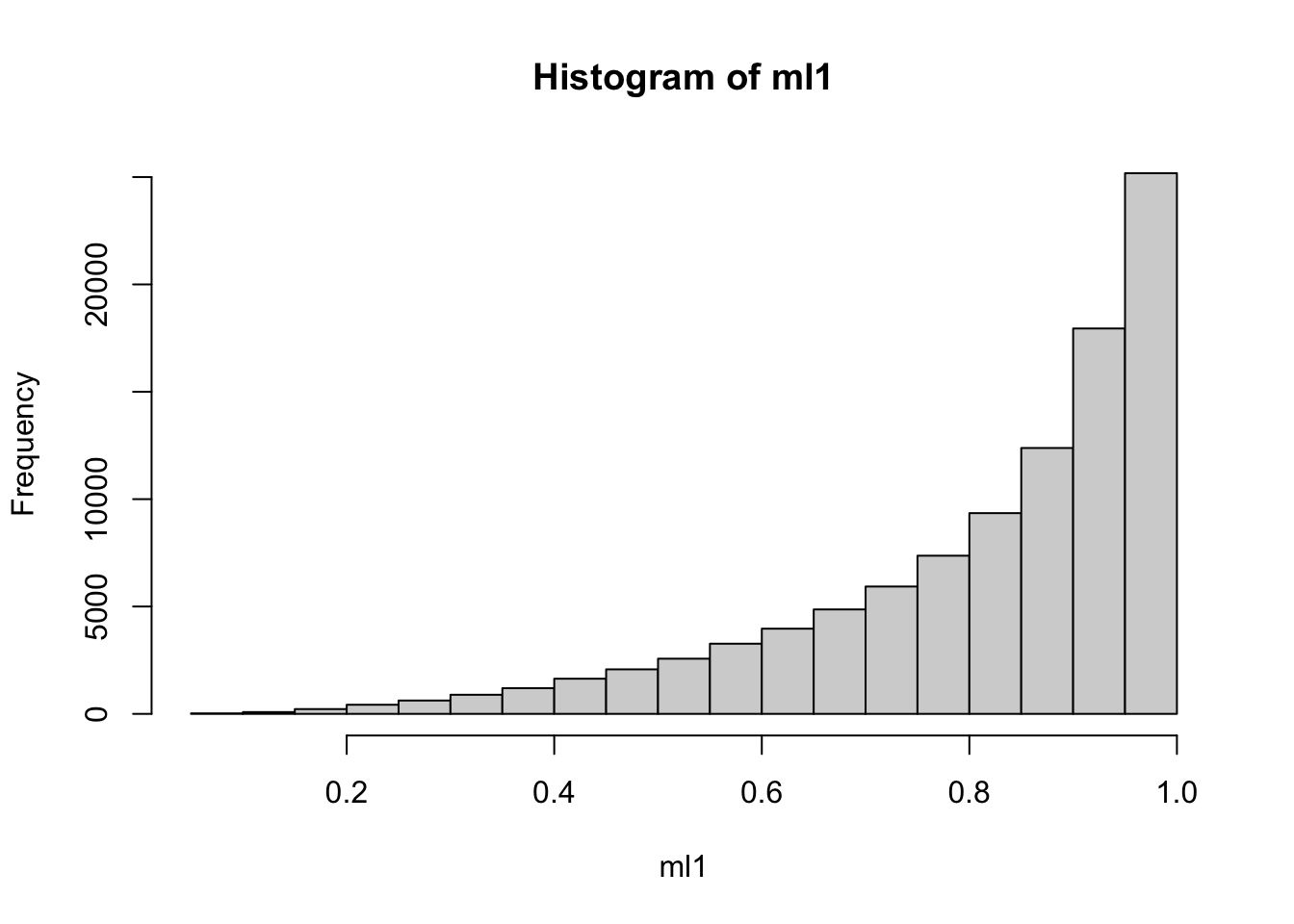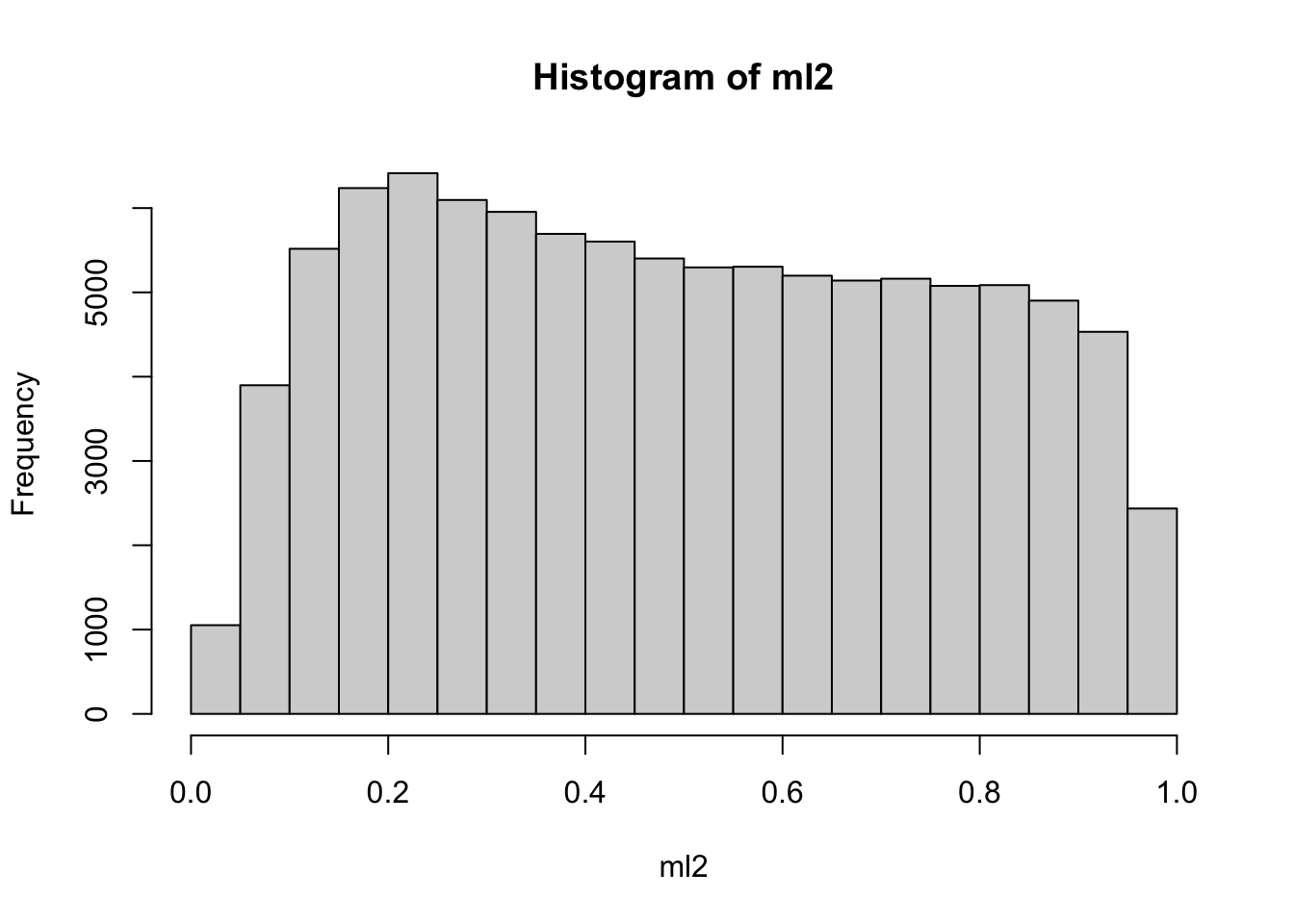# Differential susceptibility explained by interactors and mediators with different distributions

interactions
multi-ancestry
Author

Gibran Hemani

Published

June 9, 2023

## Background

What leads to different effect estimates in different populations? If the DAG is the same, then different distributions of mediators and interactions could explain different effects

``````n <- 100000

g1 <- rbinom(n, 2, 0.3)
g2 <- rbinom(n, 2, 0.3)

# The liability for the mediator
# Influenced by g (same effect in both populations)
# Influenced by some other variable that gives it a different mean in the two populations
# Now liability of mediator has different means
ml1 <- plogis(scale(g1) + rnorm(n, 2, 1))
ml2 <- plogis(scale(g2) + rnorm(n, 0, 1))

hist(ml1)````````hist(ml2)````````# Generate mediators - they have different means
m1 <- rbinom(n, 1, ml1)
m2 <- rbinom(n, 1, ml2)
mean(m1)``````
`` 0.81663``
``mean(m2)``
`` 0.49808``

Disease influenced by mediator and noise (same effects)

``````d1 <- rbinom(n, 1, plogis(m1 + rnorm(n)))
d2 <- rbinom(n, 1, plogis(m2 + rnorm(n)))

mean(d1)``````
`` 0.66026``
``mean(d2)``
`` 0.59796``

Slightly different prevalence in different populations.

The genotypic effect is now different in the two populations due to different

``glm(d1 ~ g1, family="binomial")``
``````
Call:  glm(formula = d1 ~ g1, family = "binomial")

Coefficients:
(Intercept)           g1
0.5736       0.1538

Degrees of Freedom: 99999 Total (i.e. Null);  99998 Residual
Null Deviance:      128200
Residual Deviance: 128000   AIC: 128000``````
``glm(d2 ~ g2, family="binomial")``
``````
Call:  glm(formula = d2 ~ g2, family = "binomial")

Coefficients:
(Intercept)           g2
0.2551       0.2405

Degrees of Freedom: 99999 Total (i.e. Null);  99998 Residual
Null Deviance:      134800
Residual Deviance: 134200   AIC: 134200``````

``sessionInfo()``
``````R version 4.3.0 (2023-04-21)
Platform: aarch64-apple-darwin20 (64-bit)
Running under: macOS Monterey 12.6.2

Matrix products: default
BLAS:   /Library/Frameworks/R.framework/Versions/4.3-arm64/Resources/lib/libRblas.0.dylib
LAPACK: /Library/Frameworks/R.framework/Versions/4.3-arm64/Resources/lib/libRlapack.dylib;  LAPACK version 3.11.0

locale:
 en_GB.UTF-8/en_GB.UTF-8/en_GB.UTF-8/C/en_GB.UTF-8/en_GB.UTF-8

time zone: Europe/London
tzcode source: internal

attached base packages:
 stats     graphics  grDevices utils     datasets  methods   base

loaded via a namespace (and not attached):
 htmlwidgets_1.6.2 compiler_4.3.0    fastmap_1.1.1     cli_3.6.1
 tools_4.3.0       htmltools_0.5.5   rstudioapi_0.14   yaml_2.3.7
 rmarkdown_2.22    knitr_1.43        xfun_0.39         digest_0.6.31
 jsonlite_1.8.5    rlang_1.1.1       evaluate_0.21    ``````